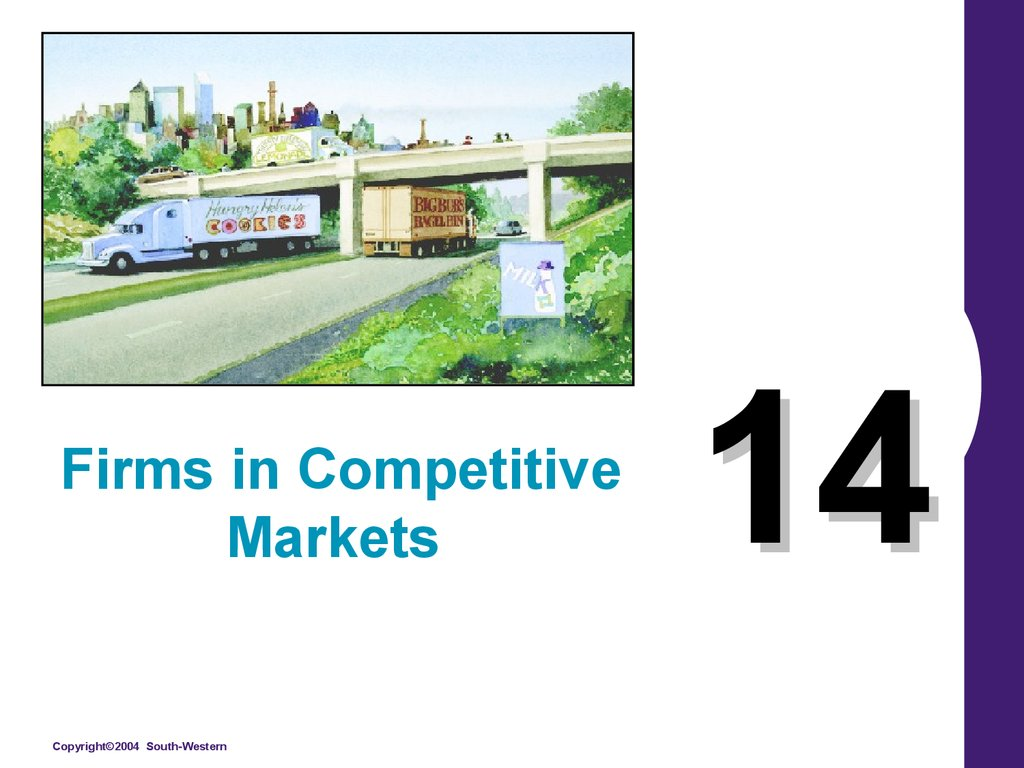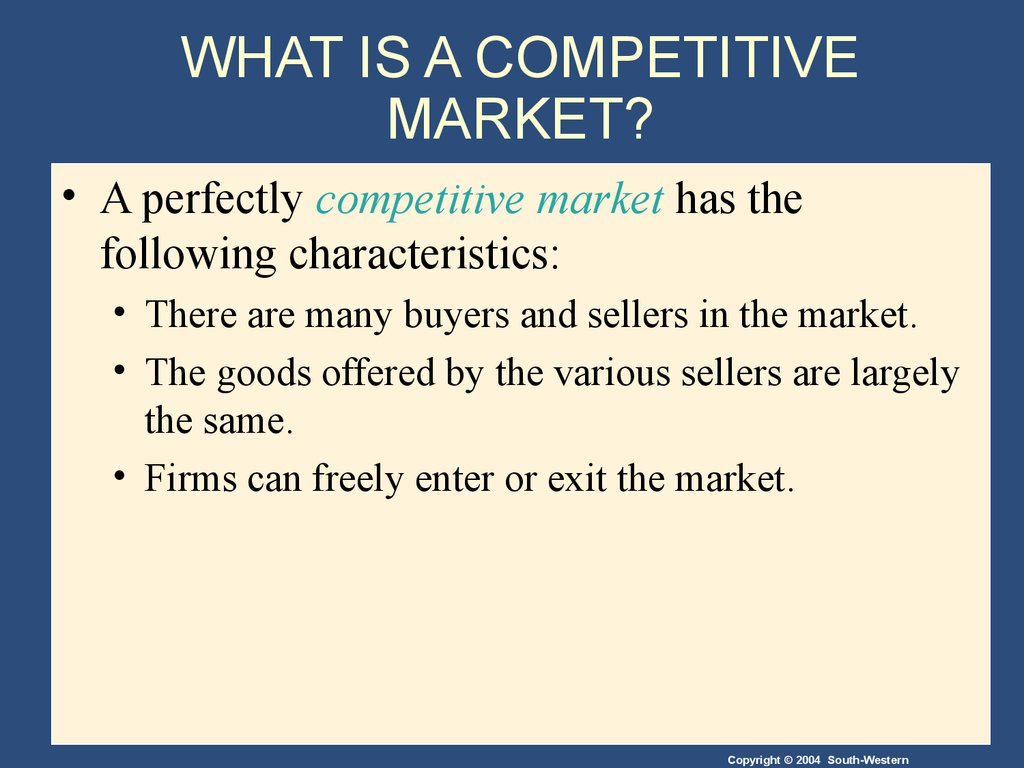# Firms in competitive markets. (Lecture 14)

## 1. 14

Firms in Competitive
Markets
14

## 2. WHAT IS A COMPETITIVE MARKET?

• A perfectly competitive market has the
following characteristics:
• There are many buyers and sellers in the market.
• The goods offered by the various sellers are largely
the same.
• Firms can freely enter or exit the market.

## 3. WHAT IS A COMPETITIVE MARKET?

• As a result of its characteristics, the perfectly
competitive market has the following
outcomes:
• The actions of any single buyer or seller in the
market have a negligible impact on the market
price.
• Each buyer and seller takes the market price as
given.

## 4. WHAT IS A COMPETITIVE MARKET?

• A competitive market has many buyers and
sellers trading identical products so that each
buyer and seller is a price taker.
• Buyers and sellers must accept the price determined
by the market.

## 5. The Revenue of a Competitive Firm

• Total revenue for a firm is the selling price
times the quantity sold.
TR = (P Q)

## 6. The Revenue of a Competitive Firm

• Total revenue is proportional to the amount of
output.

## 7. The Revenue of a Competitive Firm

• Average revenue tells us how much revenue a
firm receives for the typical unit sold.
• Average revenue is total revenue divided by the
quantity sold.

## 8. The Revenue of a Competitive Firm

• In perfect competition, average revenue equals
the price of the good.
T o ta l re v e n u e
A v e ra g e R e v e n u e =
Q u a n tity
P ric e Q u a n tity
Q u a n tity
P ric e

## 9. The Revenue of a Competitive Firm

• Marginal revenue is the change in total revenue
MR = TR/ Q

## 10. The Revenue of a Competitive Firm

• For competitive firms, marginal revenue equals
the price of the good.

## 12. PROFIT MAXIMIZATION AND THE COMPETITIVE FIRM’S SUPPLY CURVE

• The goal of a competitive firm is to maximize
profit.
• This means that the firm will want to produce
the quantity that maximizes the difference
between total revenue and total cost.

## 14. Figure 1 Profit Maximization for a Competitive Firm

Costs
and
Revenue
The firm maximizes
profit by producing
the quantity at which
marginal cost equals
marginal revenue.
MC
MC2
ATC
P = MR1 = MR2
AVC
P = AR = MR
MC1
0
Q1
QMAX
Q2
Quantity

## 15. PROFIT MAXIMIZATION AND THE COMPETITIVE FIRM’S SUPPLY CURVE

• Profit maximization occurs at the quantity
where marginal revenue equals marginal cost.

## 16. PROFIT MAXIMIZATION AND THE COMPETITIVE FIRM’S SUPPLY CURVE

When MR > MC - increase Q
When MR < MC - decrease Q
When MR = MC - Profit is maximized.

## 17. Figure 2 Marginal Cost as the Competitive Firm’s Supply Curve

Price
P2
This section of the
firm’s MC curve is
also the firm’s supply
curve.
MC
ATC
P1
AVC
0
Q1
Q2
Quantity

## 18. The Firm’s Short-Run Decision to Shut Down

• A shutdown refers to a short-run decision not to
produce anything during a specific period of
time because of current market conditions.
• Exit refers to a long-run decision to leave the
market.

## 19. The Firm’s Short-Run Decision to Shut Down

• The firm considers its sunk costs when deciding
to exit, but ignores them when deciding
whether to shut down.
• Sunk costs are costs that have already been
committed and cannot be recovered.

## 20. The Firm’s Short-Run Decision to Shut Down

• The firm shuts down if the revenue it gets from
producing is less than the variable cost of
production.
• Shut down if TR < VC
• Shut down if TR/Q < VC/Q
• Shut down if P < AVC

## 21. Figure 3 The Competitive Firm’s Short Run Supply Curve

Costs
If P > ATC, the firm
will continue to
produce at a profit.
Firm’s short-run
supply curve
MC
ATC
If P > AVC, firm will
continue to produce
in the short run.
AVC
Firm
shuts
down if
P< AVC
0
Quantity

## 22. The Firm’s Short-Run Decision to Shut Down

• The portion of the marginal-cost curve that lies
above average variable cost is the competitive
firm’s short-run supply curve.

## 23. The Firm’s Long-Run Decision to Exit or Enter a Market

• In the long run, the firm exits if the revenue it
would get from producing is less than its total
cost.
• Exit if TR < TC
• Exit if TR/Q < TC/Q
• Exit if P < ATC

## 24. The Firm’s Long-Run Decision to Exit or Enter a Market

• A firm will enter the industry if such an action
would be profitable.
• Enter if TR > TC
• Enter if TR/Q > TC/Q
• Enter if P > ATC

Costs
Firm’s long-run
supply curve
Firm
enters if
P > ATC
MC = long-run S
ATC
Firm
exits if
P < ATC
0
Quantity

## 26. THE SUPPLY CURVE IN A COMPETITIVE MARKET

• The competitive firm’s long-run supply curve is
the portion of its marginal-cost curve that lies
above average total cost.

Costs
MC
Firm’s long-run
supply curve
ATC
0
Quantity

## 28. THE SUPPLY CURVE IN A COMPETITIVE MARKET

• Short-Run Supply Curve
• The portion of its marginal cost curve that lies
above average variable cost.
• Long-Run Supply Curve
• The marginal cost curve above the minimum point
of its average total cost curve.

## 29. Figure 5 Profit as the Area between Price and Average Total Cost

(a) A Firm with Profits
Price
MC
ATC
Profit
P
ATC
P = AR = MR
0
Quantity
Q
(profit-maximizing quantity)

## 30. Figure 5 Profit as the Area between Price and Average Total Cost

(b) A Firm with Losses
Price
MC
ATC
ATC
P
P = AR = MR
Loss
0
Q
(loss-minimizing quantity)
Quantity

## 31. THE SUPPLY CURVE IN A COMPETITIVE MARKET

• Market supply equals the sum of the quantities
supplied by the individual firms in the market.

## 32. The Short Run: Market Supply with a Fixed Number of Firms

• For any given price, each firm supplies a
quantity of output so that its marginal cost
equals price.
• The market supply curve reflects the individual
firms’ marginal cost curves.

## 33. Figure 6 Market Supply with a Fixed Number of Firms

(a) Individual Firm Supply
(b) Market Supply
Price
Price
MC
Supply
\$2.00
\$2.00
1.00
1.00
0
100
200
Quantity (firm)
0
100,000
200,000 Quantity (market)

## 34. The Long Run: Market Supply with Entry and Exit

• Firms will enter or exit the market until profit is
driven to zero.
• In the long run, price equals the minimum of
average total cost.
• The long-run market supply curve is horizontal
at this price.

## 35. Figure 7 Market Supply with Entry and Exit

(a) Firm’s Zero-Profit Condition
(b) Market Supply
Price
Price
MC
ATC
P = minimum
ATC
0
Supply
Quantity (firm)
0
Quantity (market)

## 36. The Long Run: Market Supply with Entry and Exit

• At the end of the process of entry and exit,
firms that remain must be making zero
economic profit.
• The process of entry and exit ends only when
price and average total cost are driven to
equality.
• Long-run equilibrium must have firms
operating at their efficient scale.

## 37. Why Do Competitive Firms Stay in Business If They Make Zero Profit?

• Profit equals total revenue minus total cost.
• Total cost includes all the opportunity costs of
the firm.
• In the zero-profit equilibrium, the firm’s
revenue compensates the owners for the time
and money they expend to keep the business
going.

## 38. A Shift in Demand in the Short Run and Long Run

• An increase in demand raises price and quantity
in the short run.
• Firms earn profits because price now exceeds
average total cost.

## 39. Figure 8 An Increase in Demand in the Short Run and Long Run

(a) Initial Condition
Market
Firm
Price
Price
MC
ATC
P1
Short-run supply, S1
P1
A
Long-run
supply
Demand, D1
0
Quantity (firm)
0
Q1
Quantity (market)

## 40. Figure 8 An Increase in Demand in the Short Run and Long Run

(b) Short-Run Response
Market
Firm
Price
Price
Profit
MC
ATC
P2
B
P2
P1
P1
S1
A
D2
Long-run
supply
D1
0
Quantity (firm)
0
Q1
Q2
Quantity (market)

## 41. Figure 8 An Increase in Demand in the Short Run and Long Run

(c) Long-Run Response
Market
Firm
Price
Price
MC
ATC
P1
B
P2
P1
S1
S2
C
A
Long-run
supply
D2
D1
0
Quantity (firm)
0
Q1
Q2
Q3 Quantity (market)

## 42. Why the Long-Run Supply Curve Might Slope Upward

• Some resources used in production may be
available only in limited quantities.
• Firms may have different costs.

## 43. Why the Long-Run Supply Curve Might Slope Upward

• Marginal Firm
• The marginal firm is the firm that would exit the
market if the price were any lower.

## 44. Summary

• Because a competitive firm is a price taker, its
revenue is proportional to the amount of output
it produces.
• The price of the good equals both the firm’s
average revenue and its marginal revenue.

## 45. Summary

• To maximize profit, a firm chooses the quantity
of output such that marginal revenue equals
marginal cost.
• This is also the quantity at which price equals
marginal cost.
• Therefore, the firm’s marginal cost curve is its
supply curve.

## 46. Summary

• In the short run, when a firm cannot recover its
fixed costs, the firm will choose to shut down
temporarily if the price of the good is less than
average variable cost.
• In the long run, when the firm can recover both
fixed and variable costs, it will choose to exit if
the price is less than average total cost.